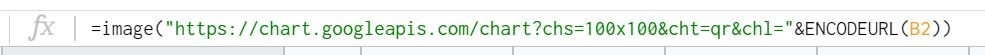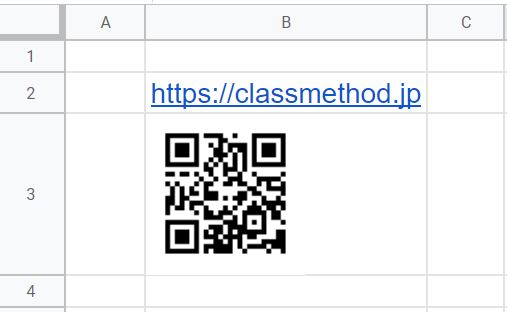この記事は公開されてから1年以上経過しています。情報が古い可能性がありますので、ご注意ください。

(和文はこちらから)

## Introduction:

In recent times, QR codes have gained popularity due to their accuracy and ease of sharing information. Now it is possible to generate QR codes using Google sheets. In an earlier post you may have noticed how to create QR codes and barcodes using Alteryx. This post explains how to create QR codes using Google Sheets.

## How to Achieve it:

Now it is possible to generate a QR code in Google Sheets using a formula. The syntax of the formula is as shown below.

``=image("https://chart.googleapis.com/chart?chs=100x100&cht=qr&chl="&ENCODEURL(A1))``

In this formula, the value A1 is to be replaced with the actual cell position which contains the target text. The 100x100 is the actual image resolution of QR code, the actual size can be modified by changing the cell width and height.

Using this formula, the sample result is as shown below.## Summary:

Using Google Sheets it is now possible to generate QR codes. This often comes handy whenever such needs arise during data analysis.